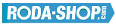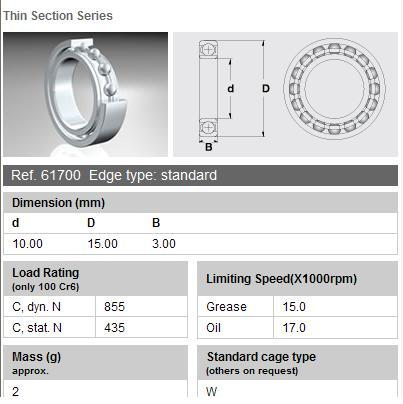# Chrome Steel BearingsChrome Steel 100Cr6(Nr.1.3505)

Manufacturer Product Image Item Name Price### 61802-2RS

inside diameter = 15
outside diameter = 24
width = 5
€4.54### 619/22-2Z

inside diameter = 22
outside diameter = 39
width = 9
€22.39### 61700

Thin Section Ball Bearing Chrome Bearing Type = Open (No Shields) Dimension d= 10.00 Dimension D= 15.00 Dimension B= 3.00 Load Rating Dynamic= 855 Load Rating Static= 435 Limiting Speed Grease(x1000 rpm)= 15.0 Limiting Speed Oil(x1000 rpm)=17.0 Cage= W Estimated Weight = 2 gr.
inside diameter = 10.00
outside diameter = 15.00
width = 3.00
€4.24### 61700

Thin Section Bearings Chrome Steel Open(No Shields) Dimension d=10.00 Dimension D=15.00 Dimension B=3.00 Dinamic Load Rating=855 Static Load Rating=435 Weight=0.002gr. Speed limit Grease=15.0 Speed limit Oil=17.0
inside diameter = 10.00
outside diameter = 15.00
width = 3.00
€4.24### 61700-2RS

Thin Section Bearings Chrome Steel Rubber Seals on Both sides Dimension d=10.00 Dimension D=15.00 Dimension B=4.00 Dinamic Load Rating=855 Static Load Rating=435 Weight=0.002gr. Speed limit Grease=15.0 Speed limit Oil=17.0
inside diameter = 10.00
outside diameter = 15.00
width = 4.00
€4.24### 61700-2Z

Thin Section Bearings Chrome Steel Metallic Shields on both sides Dimension d=10.00 Dimension D=15.00 Dimension B=4.00 Dinamic Load Rating=855 Static Load Rating=435 Weight=0.002gr. Speed limit Grease=15.0 Speed limit Oil=17.0
inside diameter = 10.00
outside diameter = 15.00
width = 4.00
€4.24### 61701

Thin Section Bearings Chrome Steel Open(No Shields) Dimension d=12.00 Dimension D=18.00 Dimension B=4.00 Dinamic Load Rating=926 Static Load Rating=530 Weight=0.004gr. Speed limit Grease=13.0 Speed limit Oil=15.0
inside diameter = 12.00
outside diameter = 18.00
width = 4.00
€4.24### 61701-2RS

Thin Section Bearings Chrome Steel Rubber Seals on Both sides Dimension d=12.00 Dimension D=18.00 Dimension B=4.00 Dinamic Load Rating=926 Static Load Rating=530 Weight=0.004gr. Speed limit Grease=13.0 Speed limit Oil=15.0
inside diameter = 12.00
outside diameter = 18.00
width = 4.00
€4.24### 61701-2Z

Thin Section Bearings Chrome Steel Metallic Shields on both sides Dimension d=12.00 Dimension D=18.00 Dimension B=4.00 Dinamic Load Rating=926 Static Load Rating=530 Weight=0.004gr. Speed limit Grease=13.0 Speed limit Oil=15.0
inside diameter = 12.00
outside diameter = 18.00
width = 4.00
€4.24### 61702

Thin Section Bearings Chrome Steel Open(No Shields) Dimension d=15.00 Dimension D=21.00 Dimension B=4.00 Dinamic Load Rating=937 Static Load Rating=582 Weight=0.004gr. Speed limit Grease=11.0 Speed limit Oil=13.0
inside diameter = 15.00
outside diameter = 21.00
width = 4.00
€4.54